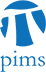## Game-theoretic Probability and its Applications

• Date: 05/26/2010
Lecturer(s):
Glenn Shafer (Rutgers University)
Location:

University of Regina

Description:

The game-theoretic framework, introduced by Vovk and myself in 2001 (www.probabilityandfinance.com), uses game theory instead of measure theory as a mathematical framework for probability. Classical theorems are proven by betting strategies that multiply a player's stake by a large factor if the theorem's prediction fails. In this talk, I will discuss the basic idea of game-theoretic probability and two important applications: (1) defensive forecasting, (2) the game-theoretic interpretation of Bayesian and Dempster-Shafer inference.

Schedule:

Time: 10:30am - 11:30am

Location: CL 125 (Classroom Building - University of Regina)

Organizers:

Cory Butz: butz@cs.uregina.ca

Other Information: